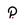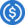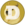# Exponential Moving Average (EMA) Definition

The exponential moving average (EMA) is an indicator that keeps a track of the price of any investment over a period of time. As its name suggests, it is a type of moving average (MA). Basically, the exponential moving average is a technical chart indicator that is used to view price trends over periods of time. Traders usually tend to watch numerous EMAs during the same time through moving average ribbons. Compared to other moving averages (MAs), the exponential moving average tends to give more significance to recent data.

These indicators are designed to view price trends throughout particular periods of time, be that 20 or 100 days.

## How to Calculate the Exponential Moving Average?

Calculating the exponential moving average is done by starting with the simple moving average (SMA) and using a multiplier. However, chart applications are an easier way to conduct the calculation since they will do the math for you. This calculation is done in three easy steps:

1. Calculate the SMA
2. Compute the multiplier needed to weigh the EMA
3. Measure the current EMA

Also Read: What is the Relative Strength Index (RSI)?

## Takeaways

• The exponential moving average (EMA) is a moving average that prioritizes the most recent data.
• It is designed to show data trends for a specific period of time.
• You can directly calculate the EMA, however, calculating it by finding the simple moving average (SMA) is recommended.

## Recent Articles

### Related Stories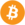Bitcoin (BTC) \$ 42,437.00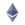Ethereum (ETH) \$ 2,889.82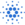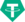Tether (USDT) \$ 1.00Binance Coin (BNB) \$ 349.02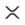XRP (XRP) \$ 0.933181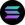Solana (SOL) \$ 137.70